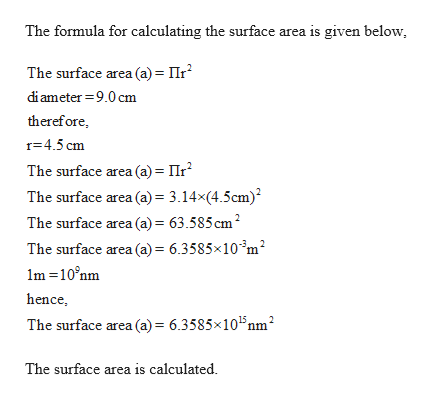Calculate the surface area in nm^2 of water contained in a watch glass with a diameter of 9.0cm. The area of a circle is calculated as A=r^2(pi)If a molecule of stearic acid covers an area of 0.21 nm^2, how many stearic acid molecules will it take to cover the surface of the watch glass in question 3.

Question

Calculate the surface area in nm^2 of water contained in a watch glass with a diameter of 9.0cm. The area of a circle is calculated as A=r^2(pi)

If a molecule of stearic acid covers an area of 0.21 nm^2, how many stearic acid molecules will it take to cover the surface of the watch glass in question 3.

Step 1

Given,

Diameter = ...help_outlineImage TranscriptioncloseThe formula for calculating the surface area is given below, The surface area (a) = IIr diameter 9.0 cm therefore r 4.5 cm The surface area (a) Ir The surface area (a) 3.14x(4.5cm)2 The surface area (a)= 63.585 cm2 The surface area (a)= 6.3585x10 m2 m 10 nm hence The surface area (a)= 6.3585x1015nm2 The surface area is calculated fullscreen

Want to see the full answer?

See Solution

Want to see this answer and more?

Our solutions are written by experts, many with advanced degrees, and available 24/7

See Solution
Tagged in

General Chemistry